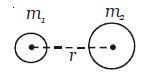# Explain Newton’s Gravitational Force Law

Special features of the law

(i) The gravitational force between two bodies is an action and reaction pair.

(ii) The gravitational force is very small in the case of lighter bodies. It is appreciable in the case of massive bodies. The gravitational force between the am and the Earth is of the order of 1027 N.

Newton suggested that gravitational force might vary inversely as the square of the distance between the bodies. He realized that this force of attraction was a case of universal attraction between any two bodies present anywhere in the universe and proposed the universal gravitational law.

The law states that every particle of matter in the universe attracts every other particle with a force which is directly proportional to the product of their masses and inversely proportional to the square of the distance between them.

Consider two bodies of masses m1 and m2 with their centres separated by a distance r. The gravitational force between them is;F α m1m2

F α 1/r2

So, F α (m1m2)/r2

Then, F = G (m1m2)/r2; where G is the universal gravitational constant.